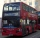# Expression of a variable from the formula + Mathematical Olympiad - math problems

#### Number of problems found: 5

• Average ageThe average age of all people at the celebration was equal to the number of people present. After the departure of one person who was 29 years old, average age was again equal to the number present. How many people were originally to celebrate?
• MO-Z5-3-66 tilesThe picture shows a square tiles with side 10 dm which is composed of four identical small rectangles and squares. Circumference of small square is five times smaller than the circumference of the entire tile. Determine the dimensions of the rectangle.
• Equilateral triangle ABCIn the equilateral triangle ABC, K is the center of the AB side, the L point lies on one-third of the BC side near the point C, and the point M lies in the one-third of the side of the AC side closer to the point A. Find what part of the ABC triangle cont
• Between two bus stopsWanda lives between two bus stops at three-eighths of their distance. He started the house today and found that whether he was running to one or the other stop, he would have arrived at the bus stop. The average bus speed is 60 km/h. What is the average s
• MO SK/CZ Z9–I–3John had the ball that rolled into the pool, and it swam in the water. Its highest point was 2 cm above the surface. The diameter of the circle that marked the water level on the surface of the ball was 8 cm. Find the diameter of John ball.

We apologize, but in this category are not a lot of examples.
Do you have an interesting mathematical word problem that you can't solve it? Submit a math problem, and we can try to solve it.

We will send a solution to your e-mail address. Solved examples are also published here. Please enter the e-mail correctly and check whether you don't have a full mailbox.

Please do not submit problems from current active competitions such as Mathematical Olympiad, correspondence seminars etc...

Expression of a variable from the formula - math word problems. Mathematical Olympiad - math word problems.